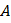# The equivalent resistance and potential difference betweenandfor the circuit is respectivelya)b)c)d)## Question ID - 150351 :- The equivalent resistance and potential difference betweenandfor the circuit is respectivelya)b)c)d)3537

 (a) The equivalent resistance betweenandisorNow the equivalent resistance betweenandasandare connected in series, soNow by ohm’s law, potential difference betweenandis given byA wire of resistance 18is divided into three equal parts. These parts are connected in side of triangle, the equivalent resistance of any two corners of triangle will be a)b)c)d)